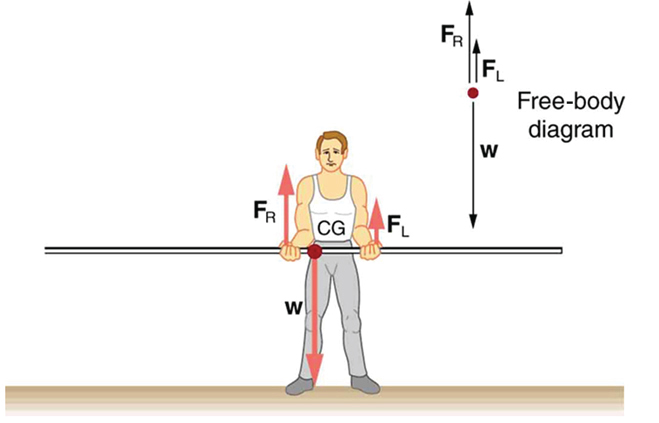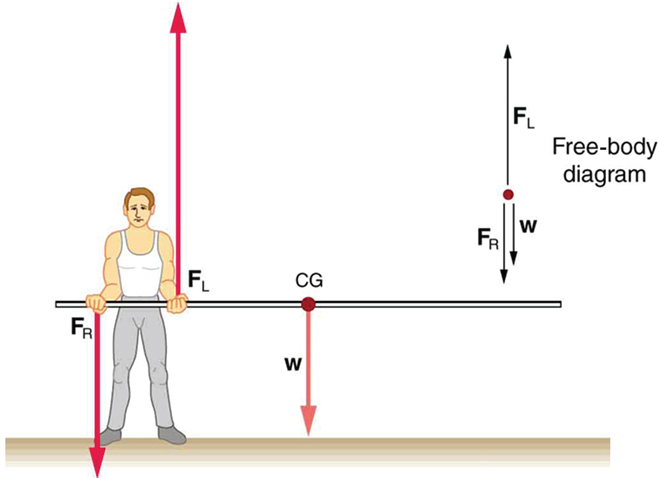# 9.4 Applications of statics, including problem-solving strategies  (Page 2/3)

 Page 2 / 3

Similar observations can be made using a meter stick held at different locations along its length.A pole vaulter is holding a pole horizontally with both hands. The center of gravity is near his right hand.A pole vaulter is holding a pole horizontally with both hands. The center of gravity is to the left side of the vaulter.

If the pole vaulter holds the pole as shown in [link] , the situation is not as simple. The total force he exerts is still equal to the weight of the pole, but it is not evenly divided between his hands. (If ${F}_{L}={F}_{R}$ , then the torques about the cg would not be equal since the lever arms are different.) Logically, the right hand should support more weight, since it is closer to the cg. In fact, if the right hand is moved directly under the cg, it will support all the weight. This situation is exactly analogous to two people carrying a load; the one closer to the cg carries more of its weight. Finding the forces ${F}_{L}$ and ${F}_{R}$ is straightforward, as the next example shows.

If the pole vaulter holds the pole from near the end of the pole ( [link] ), the direction of the force applied by the right hand of the vaulter reverses its direction.

## What force is needed to support a weight held near its cg?

For the situation shown in [link] , calculate: (a) ${F}_{R}$ , the force exerted by the right hand, and (b) ${F}_{L}$ , the force exerted by the left hand. The hands are 0.900 m apart, and the cg of the pole is 0.600 m from the left hand.

Strategy

[link] includes a free body diagram for the pole, the system of interest. There is not enough information to use the first condition for equilibrium $\text{(net}\phantom{\rule{0.25em}{0ex}}F=0$ ), since two of the three forces are unknown and the hand forces cannot be assumed to be equal in this case. There is enough information to use the second condition for equilibrium $\text{(net}\phantom{\rule{0.25em}{0ex}}\tau =0\text{)}$ if the pivot point is chosen to be at either hand, thereby making the torque from that hand zero. We choose to locate the pivot at the left hand in this part of the problem, to eliminate the torque from the left hand.

Solution for (a)

There are now only two nonzero torques, those from the gravitational force ( ${\tau }_{\text{w}}$ ) and from the push or pull of the right hand ( ${\tau }_{R}$ ). Stating the second condition in terms of clockwise and counterclockwise torques,

$\text{net}\phantom{\rule{0.25em}{0ex}}{\tau }_{\text{cw}}=\text{–net}\phantom{\rule{0.25em}{0ex}}{\tau }_{\text{ccw}}\text{.}$

or the algebraic sum of the torques is zero.

Here this is

${\tau }_{R}={\mathrm{–\tau }}_{\text{w}}$

since the weight of the pole creates a counterclockwise torque and the right hand counters with a clockwise torque. Using the definition of torque, $\tau =\text{rF}\phantom{\rule{0.25em}{0ex}}\text{sin}\phantom{\rule{0.25em}{0ex}}\theta$ , noting that $\theta =90º$ , and substituting known values, we obtain

$\left(0\text{.}\text{900 m}\right)\left({F}_{R}\right)=\left(0\text{.600 m}\right)\left(\mathit{mg}\right)\text{.}$

Thus,

$\begin{array}{lll}{F}_{R}& =& \left(0.667\right)\left(\text{5.00 kg}\right)\left(9.80\phantom{\rule{0.25em}{0ex}}{\text{m/s}}^{2}\right)\\ & =& \text{32.7 N.}\end{array}$

Solution for (b)

The first condition for equilibrium is based on the free body diagram in the figure. This implies that by Newton’s second law:

${F}_{L}+{F}_{R}–\text{mg}=0$

From this we can conclude:

${F}_{L}+{F}_{R}=w=\text{mg}$

Solving for ${F}_{L}$ , we obtain

$\begin{array}{lll}{F}_{L}& =& \mathit{mg}-{F}_{R}\\ & =& \mathit{mg}-\text{32}\text{.}7 N\\ & =& \left(\text{5.00 kg}\right)\left(\text{9.80}\phantom{\rule{0.25em}{0ex}}{\text{m/s}}^{2}\right)-\text{32.7 N}\\ & =& \text{16.3 N}\end{array}$

Discussion

${F}_{L}$ is seen to be exactly half of ${F}_{R}$ , as we might have guessed, since ${F}_{L}$ is applied twice as far from the cg as ${F}_{R}$ .

If the pole vaulter holds the pole as he might at the start of a run, shown in [link] , the forces change again. Both are considerably greater, and one force reverses direction.

## Take-home experiment

This is an experiment to perform while standing in a bus or a train. Stand facing sideways. How do you move your body to readjust the distribution of your mass as the bus accelerates and decelerates? Now stand facing forward. How do you move your body to readjust the distribution of your mass as the bus accelerates and decelerates? Why is it easier and safer to stand facing sideways rather than forward? Note: For your safety (and those around you), make sure you are holding onto something while you carry out this activity!

## Phet explorations: balancing act

Play with objects on a teeter totter to learn about balance. Test what you've learned by trying the Balance Challenge game.

## Summary

• Statics can be applied to a variety of situations, ranging from raising a drawbridge to bad posture and back strain. We have discussed the problem-solving strategies specifically useful for statics. Statics is a special case of Newton’s laws, both the general problem-solving strategies and the special strategies for Newton’s laws, discussed in Problem-Solving Strategies , still apply.

## Conceptual questions

When visiting some countries, you may see a person balancing a load on the head. Explain why the center of mass of the load needs to be directly above the person’s neck vertebrae.

## Problems&Exercises

To get up on the roof, a person (mass 70.0 kg) places a 6.00-m aluminum ladder (mass 10.0 kg) against the house on a concrete pad with the base of the ladder 2.00 m from the house. The ladder rests against a plastic rain gutter, which we can assume to be frictionless. The center of mass of the ladder is 2 m from the bottom. The person is standing 3 m from the bottom. What are the magnitudes of the forces on the ladder at the top and bottom?

In [link] , the cg of the pole held by the pole vaulter is 2.00 m from the left hand, and the hands are 0.700 m apart. Calculate the force exerted by (a) his right hand and (b) his left hand. (c) If each hand supports half the weight of the pole in [link] , show that the second condition for equilibrium $\text{(net}\phantom{\rule{0.25em}{0ex}}{\tau }_{}=\text{0)}$ is satisfied for a pivot other than the one located at the center of gravity of the pole. Explicitly show how you follow the steps in the Problem-Solving Strategy for static equilibrium described above.

what is Andromeda
what is velocity
displacement per unit time
Murlidhar
the ratec of displacement over time
Jamie
the rate of displacement over time
Jamie
the rate of displacement over time
Jamie
did you need it right now
Pathani
up to tomorrow
Santosh
i need a description and derivation of kinetic theory of gas
Santosh
pls the sum of change in kinetic and potential energy is always what ?
Faith
i need a description and derivation of kinetic theory of gas
Santosh
did you need it right now
Pathani
A few grains of table salt were put in a cup of cold water kept at constant temperature and left undisturbed. eventually all the water tasted salty. this is due to?
Aunt Faith,please i am thinking the dissolution here from the word "solution" exposed the grains of salt to be dissolved in the water.Thankyou
Junior
Junior
Aunt Faith,please i am thinking the dissolution here from the word "solution" exposed the grains of salt to be dissolved in the water.Thankyou
Junior
it is either diffusion or osmosis. just confused
Faith
due to solvation....
Pathani
what is solvation pls
Faith
water molecule surround the salt molecules . solute solute attraction break in the same manner solvent solvent interaction also break. as a result solute and solvent attraction took place.
Pathani
okay thanks
Faith
my pleasure
Pathani
what is solvation pls
Faith
water act as a solvent and salt act as solute
Pathani
okay thanks
Faith
its ok
Pathani
due to solvation....
Pathani
water molecule surround the salt molecules . solute solute attraction break in the same manner solvent solvent interaction also break. as a result solute and solvent attraction took place.
Pathani
what is magnetism
physical phenomena arising from force caused by magnets
is the phenomenon of attracting magnetic substance like iron, cobalt etc.
Faith
what is heat
Heat is a form of energy where molecules move
saran
topic-- question
Salman
I know this is unrelated to physics, but how do I get the MCQs and essay to work. they arent clickable.
20cm3 of 1mol/dm3 solution of a monobasic acid HA and 20cm3 of 1mol/dm3 solution of NaOH are mixed in a calorimeter and a temperature rise of 274K is observed. If the heat capacity of the calorimeter is 160J/K, calculate the enthalpy of neutralization of the acid.(SHCw=4.2J/g/K) Formula. (ms*cs+C)*T
why is a body moving at a constant speed able to accelerate
20cm3 of 1mol/dm3 solution of a monobasic acid HA and 20cm3 of 1mol/dm3 solution of NaOH are mixed in a calorimeter and a temperature rise of 274K is observed. If the heat capacity of the calorimeter is 160J/K, calculate the enthalpy of neutralization of the acid.(SHCw=4.2J/g/K) Formula. (ms*cs+C)*T
Lilian
because it changes only direction and the speed is kept constant
Justice
Why is the sky blue...?
It's filtered light from the 2 forms of radiation emitted from the sun. It's mainly filtered UV rays. There's a theory titled Scatter Theory that covers this topic
Mike
A heating coil of resistance 30π is connected to a 240v supply for 5min to boil a quantity of water in a vessel of heat capacity 200jk. If the initial temperature of water is 20°c and it specific heat capacity is 4200jkgk calculate the mass of water in a vessel
A thin equi convex lens is placed on a horizontal plane mirror and a pin held 20 cm vertically above the lens concise in position with its own image the space between the undersurface of d lens and the mirror is filled with water (refractive index =1•33)and then to concise with d image d pin has to
Be raised until its distance from d lens is 27cm find d radius of curvature
Azummiri
what happens when a nuclear bomb and atom bomb bomb explode add the same time near each other
A monkey throws a coconut straight upwards from a coconut tree with a velocity of 10 ms-1. The coconut tree is 30 m high. Calculate the maximum height of the coconut from the top of the coconut tree? Can someone answer my question
v2 =u2 - 2gh 02 =10x10 - 2x9.8xh h = 100 ÷ 19.6 answer = 30 - h.
Ramonyai
why is the north side is always referring to n side of magneticByByByBy RhodesByByBy David Martin